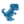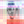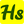0%
0%

# Digital Ammeter Using Arduino

In this project, we are going to measure the current using ohm's law.

Similar projects worth following
544 views
This project describes how to measure the current using ohm's law.

As we all are aware of ohm's law, which states that

V=IR

Where V= voltage in volt (v)

I= Current in Ampere (A)

R= Resistance in ohm (Ω)

To find I we have to use I= V/R

This Ammeter circuit contains a resistor and LED as a load. The resistor is attached in series to the LED that current flows via the load and voltage drops are discovered from the resistor.

In the ADC of Arduino that modifies the voltage into 10-bit resolution numbers from 0-1023. So we require to modify it in voltage value bu utilizing the programming.

The value of voltage from the ADC of Arduino is ranged between 0-1023 and the reference voltage is ranges between 0-5v. From the above voltage value and by dividing it with 22 ohm we can calculate current. Here we have utilized the value of 22ohm resistor as current sensor.

• 1 × Arduino UNO & Genuino UNO
• 1 × Single Turn Potentiometer- 10k ohms
• 1 × Resistor, 22 ohm
• 1 × LCD - 16x2
• 1 × LED

• 1
Run a Program

#include
LiquidCrystal lcd (7,8,9,10,11,12);

void setup() {
// put your setup code here, to run once:
Serial.begin(9600);
lcd.begin(16,2);
lcd.clear();
}

void loop() {
// put your main code here, to run repeatedly:

int subraction_value =(voltage_value0 - voltage_value1) ;
float temp_val = (subraction_value*0.00488);

float current_value = (temp_val/22);
Serial.print(current_value);
lcd.setCursor(0,0);
lcd.print("current value=");
lcd.setCursor(0,1);
lcd.print (current_value);
lcd.print("A");
delay(1000);
}

Share

## Similar Projects

Project Owner Contributor

### DIY Arduino Miliohmmeter for measure low value resmircemk

Project Owner Contributor

### Spin/Drive: Automatic potentiometer tracerBen Holmes

Project Owner Contributor

### Arduino based simple Watt-meter shieldhalfstudents.in

# Does this project spark your interest?

Become a member to follow this project and never miss any updates